﻿ 帮助

moudle=启用背景模块化设置
------------以下属性在moudle=1时才生效---------------
vertical=1(竖排显示)
alignment=1(对齐方式0,1,2)
fitWidth=0(关闭文字大小自适应)
round=5(圆角半径)
bgcolor=#d86543(背景颜色)
bdcolor=#ffffff(边框颜色,如果你设置了边框颜色请删除corners属性,否则圆角边框会显示不完全)
frame=0/4/20/10(左右/上下/宽/高)
corners=0/1/2/3(单独指定圆角 左上,右上 左下 右下)
----------------------------------------------------
SP_STEPS 每日运动步数
SP_FLOORS 每日上下楼层
SP_DISTANCE 每日运动里程
a(新版本改为了TM_A)=凌晨---深夜
ca(新版本改为了TM_CA)=中国古代时间 子时 卯时 亥时 等
E=星期一至日 例如:你设置为"礼拜E",今天是星期二的话将显示为:礼拜二.
CCD=农历年 CCM=农历月 CCD=农历日 例如:你设置为"今天是CCD"那么将显示为:今天是初二。
/n=换行符 内容需要换行使用此字符
text=格式化字符串 如果你需要让字符带有格式(大小,颜色等)请把它放在这里
size=字体大小 定义()内text字段内容的文字大小
color=字体颜色 定义()内text字段内容的颜色
bold=字体加粗 定义()内text字段内容是否加粗,blod=1为加粗其它都不加粗

`注意事项`

1.如果你的代码在某些app[如:支付宝]显示错乱,请将以下代码复制到你代码的最前面.

2.上下位置调整:使用offsety=数字(可以是负数或小数).

3.左右位置调整:用占位符(text=.....,size=10)增减字符数或者修改size大小(支持小数)

4.iOS13用户请将时间模块透明度改为1调整模块大小和位置以免挡住了返回按键,导致触摸不灵

`模块代码1`Module Size:80,55 Spaces Between:7.5 Alignment:1

`模块代码2`Module Size:80,55 Spaces Between:7.5 Alignment:1

`模块代码3`Module Size:80,55 Spaces Between:7.5 Alignment:1

`模块代码4`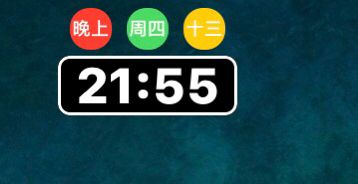Module Size:80,50 Spaces Between:12 Alignment:1

`模块代码5`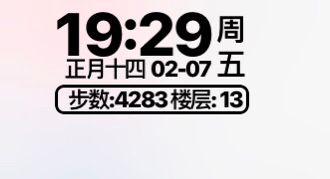Module Size:80,55 Spaces Between:8.5 Alignment:1

`模块代码6`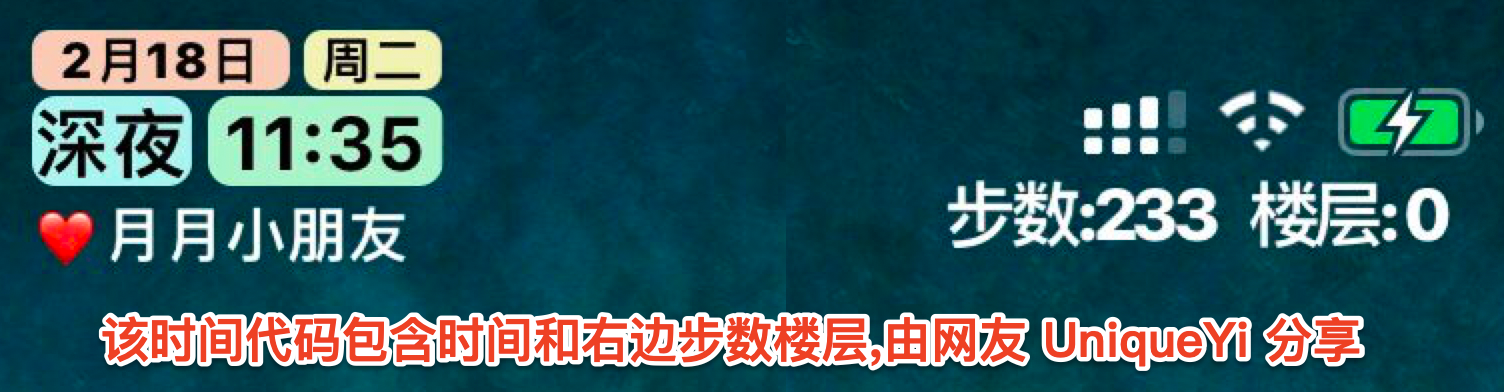Module Size:355,50 Spaces Between:7 Alignment:0

`模块代码7`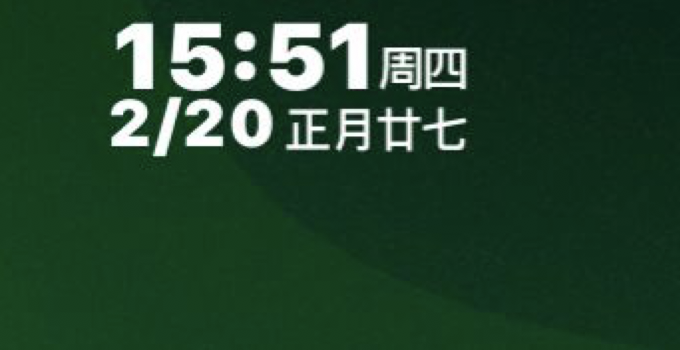Module Size:80,50 Spaces Between:10 Alignment:1

`普通代码1`
(text=HH:mm,size=16) (text=周E,size=9,bold=1,color=#ff5951)/n(text=MM,size=16)(text=/,size=8)(text=dd,size=16) (text=CCD,size=9,bold=1,color=#23ABFF)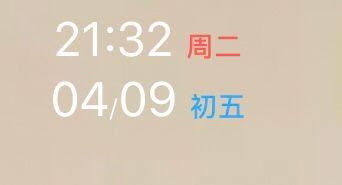HH:mm=21:32 这里只设置了size字段代码字体大小为16,如果你想21:32大点就增加小点就减小

MM=04 MM代表的是当前的月份,如果你不想要前面的0改为M即可显示为:4 后面size是它的大小,当然你也可以增加其它属性.给它上色或者加粗等
/ 这里单独给这个符号设置了一个size属性让它看起来很小,当然你也可以增加其它属性.给它上色或者加粗等
dd=09 dd代表的是当前几号今天是9号所以显示09如果你只想显示9那么改为d即可
CCD=初五 如前面所说CCD代码的是农历日,今天是初五所以就直接显示初五了

`普通代码2`
(text=HH:mm,size=14,bold=1) (text=礼拜E,size=9,bold=1)/n(text=MM月dd日,size=13,bold=1) (text=CCD,size=9,bold=1)`普通代码3`
(text=HH:mm,size=16) (text=a,size=9,bold=1) /n(text=MM月dd日,size=9) (text=CCMCCD,size=9,bold=1)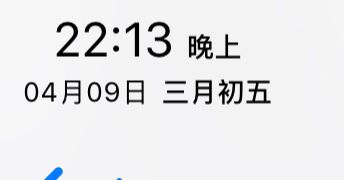`演示4(最前面加7个空格,并且更改行间距为16,换行一定要加换行符/n)`
(text=周E CCD,size=8,bold=1)/n(text=dd,size=26,bold=1,color= #ff5951)(text=HH:mm,size=13,bold=1)